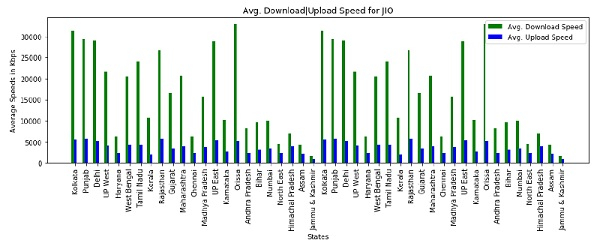# Analysing Mobile Data Speeds from TRAI with Pandas in Python

PythonServer Side ProgrammingProgramming

In this tutorial, we are going to analyze the mobile data speeds using the pandas package. Download the mobile speeds from the TRAI official website. Steps to download the file.

## Algorithm

1. Go to the [TRAI](https://myspeed.trai.gov.in/ ) website.
2. Scroll down to the end of the page.
3. You will find mobile speed data for different months.
4. Download the September mobile data speeds.

Let's see the columns in the CSV file.

• Network Name

• Network Technology

• Type Of Test

• Speed

• Signal Strength

• State

We need pandas, numpy, matplotlib libraries. Let's start the coding to analyze the data.

## Example

# importing requires libraries
import pandas as pd
import numpy as np
import matplotlib.pyplot as plot
# constants
DATASET = 'sept19_publish.csv'
NETWORK_NAME = 'JIO'
# lists to store the values
states = []
operators = []
# importing the dataset using pandas
# assigning column names for easy access
data_frame.columns = ['Network', 'Technology', 'Type Of Test', 'Speed', 'Signal Str
ength', 'State']
# getting unique states and operators from the dataset
unique_states = data_frame['State'].unique()
unique_operators = data_frame['Network'].unique()
print(unique_states)
print()
print(unique_operators)

## Output

If you run the above program you will get the following result.

['Kolkata' 'Punjab' 'Delhi' 'UP West' 'Haryana' nan 'West Bengal'
'Tamil Nadu' 'Kerala' 'Rajasthan' 'Gujarat' 'Maharashtra' 'Chennai'
'Mumbai' 'North East' 'Himachal Pradesh' 'Assam' 'Jammu & Kashmir']
['JIO' 'AIRTEL' 'VODAFONE' 'IDEA' 'CELLONE' 'DOLPHIN']

Continuation...

# getting the data related to one network that we want
# we already declared the network previously
# this filtering the data
JIO = data_frame[data_frame['Network'] == NETWORK_NAME]
# iterating through the all states
for state in unique_states:
# getting all the data of current state
current_state = JIO[JIO['State'] == state]
# getting upload speed from the current_state
# checking if the averages or nan or not
# assigning zeroes to the both speeds
else:
# appending state if the values are not nan to plot
states.append(state)
print(f'{state}: Download Avg. {download_speed_avg:.3f} Upload Avg. {upload _speed_avg:.3f}')

## Output

If you run the above code you will get the following result.

Kolkata: Download Avg. 31179.157 Upload Avg. 5597.086
Jammu & Kashmir: Download Avg. 1665.425 Upload Avg. 802.925

Continuation...

# plotting the graph'
fix, axes = plot.subplots()
# setting bar width
bar_width = 0.25
# rearranging the positions of states
re_states = np.arange(len(states))
# setting the width and height
plot.figure(num = None, figsize = (12, 5))
Speed')
plot.bar(re_states + bar_width, upload_speeds, bar_width, color='b', label='Avg. Up
# title of the graph
# x-axis label
plot.xlabel('States')
# y-axis label
plot.ylabel('Average Speeds in Kbps')
# the label below each of the bars,
# corresponding to the states
plot.xticks(re_states + bar_width, states, rotation = 90)
# draw the legend
plot.legend()
# make the graph layout tight
plot.tight_layout()
# show the graph
plot.show()

## Output

If you run the above graph you will get the following graph.## Conclusion

You can plot different graphs based on your needs. Play with the dataset by plotting different graphs. If you have any doubts regarding the tutorial, mention them in the comment section.